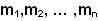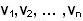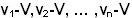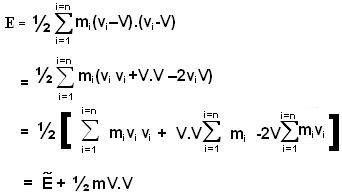## Friday, May 9, 2008

### Irodov Problem 1.148

Let the particles in the system have masses, where n is the number of particles in
the system.

The total mass of the system is, so,Let the velocities of the particles with respect to the center of mass of the system of particles be. The kinetic energy of the system as seen from the center of mass isand so,With respect to a frame fixed on the center of mass, its velocity is zero. In other words,As seen from the frame K which moves with a velocity V with respect to the center of mass, the particles have velocities,. Thus, the total kinetic energy as seen of the system of particles as seen from K is given by,